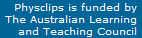# Spectrum, harmonics and timbre

Spectrum and envelope strongly influence timbre. Harmonic spectra are discussed here: the effect of envelope on timbre (often stronger than that of spectrum) has its own page. This is a background page to the multimedia chapter Quantifying Sound.

### The harmonic series

The set of frequencies f, 2f, 3f, 4f etc is the harmonic series. f is called the fundamental, 2f the second harmonic, 3f the third harmonic, etc. In general, they don't have the same amplitude. Let's hear the first six in the series, recording the amplitude of each on a separate graph.

The harmonic series can arise in one of two (or more) ways. First, some systems have resonances whose frequencies lie at or close to the harmonic series. Examples are a very thin, flexible, uniform string between two fixed ends, air in a very thin cylindrical pipe or the infinite square well in quantum mechanics. (See waves and strings and pipes and harmonics for examples.)

Second, oscillations in nonlinear systems are often periodic, and the spectrum of a periodic function (as we'll see below) is an harmonic series.

Now let's look at that series again, adding them up as we go, to make the function plotted in the graph below, which is what we'll hear, too.

Now we have a meaning for the graph or histogram we plotted in the first example. Each bar on that graph represents the amplitude of the component at that particular frequency. This is the magnitude spectrum of the black curve (and the sound we heard): you can think of it as a recipe for putting the black curve or that sound together: 'Take this much of the fundamental, this much of the second harmonic, etc'.

Notice that, after one complete cycle of the fundamental, the 2f component has had exactly two cycles, the 3f three cycles, etc. Consequently, they are all 'ready' to start again in phase. This means that, after one period of the fundamental, the function with this spectrum repeats itself exactly. A harmonic series makes a periodic signal. The reverse is also true: a periodic wave has a harmonic spectrum.

In this diagram, we've shown only the amplitude or magnitude of the harmonics and not their relative phases, which are zero in this example. Strictly speaking, the spectrum includes both. Phase is often omitted in sound spectra, in part because our ears are not very sensitive to relative phase.

### Chord or single note? Harmonic fusion

As we add waves in the harmonic series, we probably hear a chord being formed. However, if we then take that synthesised sound, with frequency f, and mutliply all the frequencies by the same constant, will we hear a series of moving chords, or will we hear a complex tone?

If you thought that the last little melody sounded like a tune made up of single notes, then the spectrum we saw above is the spectrum of the first of those notes.

In this spectrum, the amplitudes have the ratio 1 : 1/n : 1/n2 :  1/n3 etc, and the relative phases of the sine waves were all zero. If continued to very high frequencies, this 'recipe' or spectrum makes a sawtooth wave. Each octave, the amplitude decreases by 6 dB or a factor of two (1 : 1/n : 1/n2 :  1/n4 etc) so we could say that this wave has a spectral envelope of 6 dB/octave. If we plot the amplitude on a dB scale, the points on the spectrum would lie on a straight line with a slope of − 6 dB/octave.

### MoreThis work is licensed under a Creative Commons License.